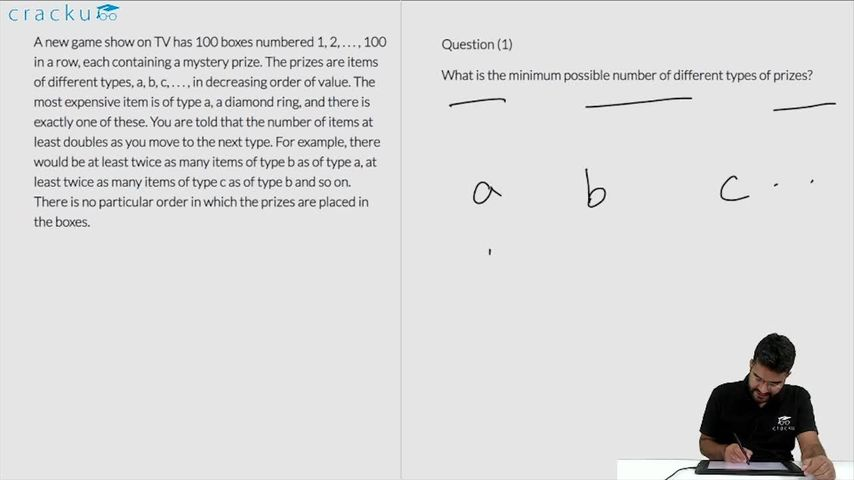### CAT 2019 Question Paper (Slot 1) Question 64

Instructions

A new game show on TV has 100 boxes numbered 1, 2, . . . , 100 in a row, each containing a mystery prize. The prizes are items of different types, a, b, c, . . . , in decreasing order of value. The most expensive item is of type a, a diamond ring, and there is exactly one of these. You are told that the number of items at least doubles as you move to the next type. For example, there would be at least twice as many items of type b as of type a, at least twice as many items of type c as of type b and so on. There is no particular order in which the prizes are placed in the boxes.

Question 64

# What is the maximum possible number of different types of prizes?

Solution

It is given that the most expensive item is a diamond ring of type a and there is exactly one of these. Since the number of items of type b should be at least twice of that of a and the number of items of type c should be at least twice of that of b and so on. So the maximum number of different types of items of a, b and c will be obtained when a=1, b=2, c=4, d=8, e=16, f=69. Hence the maximum number of different types of items will be 6.

If the number of items is 7, then the minimum number of prizes should be 1+2+4+8+16+32+64=127 which is more than 100.

### View Video Solution• All Quant CAT Formulas and shortcuts PDF
• 30+ CAT previous papers with solutions PDF